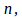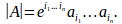## The Determinant of a Matrix

The determinant of the matrix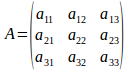can be written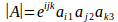where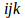is any permutation of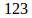and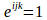if the permutation is even and -1 if the permutation is odd, and matching raised and lower indices indicate summation.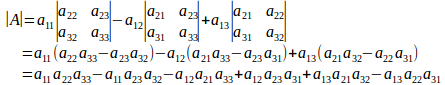In the last line 123,231,312 are even, and are added.

132, 213, 321 are odd and are subtrated.

This result can be generalised.

For a square matrix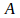of order